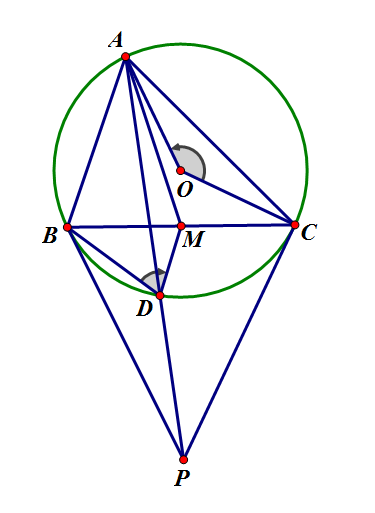# Have You Heard Of Symmedian?

Geometry Level 4A triangle $ABC$ is inscribed inside a circumcircle with center $O$. Draw the median $AM$ of the circle. Now, draw the tangents of the circle $O$ at $B$ and $C$, and they cut at point $P$. $AP$ cuts the circle $O$ at $D$. If $\angle BDM = 27^\circ$, find $\angle AOC$ in degrees.

Clarification: For anyone who doesn't know what symmedian is, it's just the reflection of the median on bisector. For example, for triangle $ABC$ with median $AM$ and bisector $AI,$ if you draw the reflection $AD$ of $AM$ on $AI,$ then $AD$ is the symmedian.

×

Problem Loading...

Note Loading...

Set Loading...